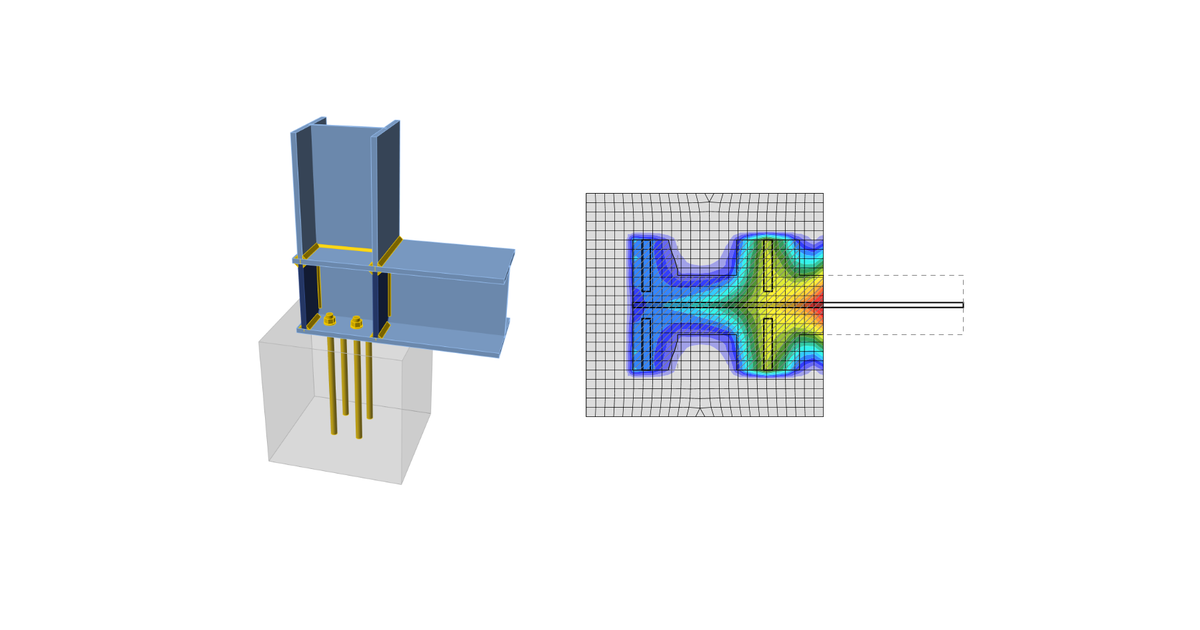### Choose language# Code-check of concrete block according to Russian standards

$$Concrete in compressionConcrete in compression under the base plate is checked according to SP 63.13330.2012, Cl. 8.1.44 – Calculation of reinforced concrete elements for local compression:$N \le \psi R_{b,loc} A_{b,loc}$where: N – local compressive force from an external load ψ – factor equal to 0.75 in case of non-uniform distribution of the local load across the bearing surface Rb,loc = φb Rb – design compressive resistance of concrete in case of the local impact of compressive force $$\varphi_b = 0.8 \sqrt{\frac{A_{b,max}}{A_{b,loc}}}$$ and 1.0 ≤ φb ≤ 2.5 – concentration factor taking into account the triaxial stress in concrete Rb = Rbn / γb – design value of concrete axial compressive resistance Rbn – normative axial compressive resistance of concrete γb = 1.3 – reliability factor for concrete under compression; editable in Code setup Ab,loc – application area of the compressive force (bearing area surface) determined by finite element method as area in contact between the base plate and concrete block Ab,max – maximum design area established based on the following rules: gravity centres of areas Ab,loc and Ab,max coincide maximum design area is geometrically similar to the application area; the slopes are 1 vertical to 2 horizontal. Transfer of shearThe shear action at the base plate is assumed to be transferred from the column to the concrete foundation by: Friction between base plate and concrete / grout Shear lug Anchor bolts AnchorsThe tensile forces in anchors include prying forces and are determined by finite element analysis.Anchors are not checked in the software.$$